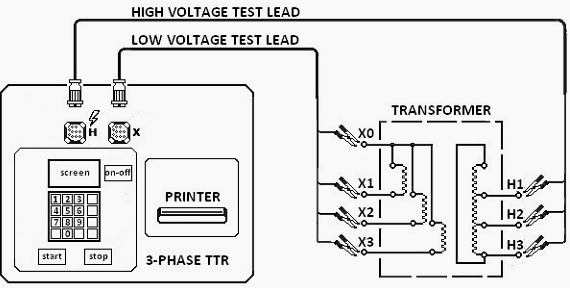# Transformer Voltage and Turn Ratio Testing

Transformer Ratio Test

Each transformer before operating will be approved through some tests as type test, special test, routine test.

In which, transformer ration test is an important type test of transformer.

The performance of a transformer is largely subject to the voltage ratio of the transformer the perfection of specific turns. So, the transformer ration test is an essential type of test of the transformer. It is recommended to apply the voltage only in the high voltage winding in order to avoid unsafe voltage.

Ratio Test of Transformer and Check of Phase Displacement

Actually, the turn ratio is equal to the no-load voltage ratio of the transformer.

The procedure of Transformer Ratio Test

1. First, keep the tap changer of the transformer in the lowest position and LV terminals open.
2. Then 3-phase 415 V supply is applied on HV terminals. Measure the voltages applied on each phase (Phase-phase) on HV and induced voltages at LV terminals simultaneously.
3. After the voltages at HV and LV terminals are measured, raise the tap changer of the transformer by one position and repeat the test.
4. Repeat the same for each of the tap positions separately.

The portable transformer turns ratio (TTR) meter is used to perform the above transformer ratio test. They have an in-built power supply, with the used very low voltages, such as 8-10 V and 50 Hz. The LV and HV windings of one phase of a transformer are linked to the instrument, and the internal bridge elements are changed to produce a null indication on the detector.

Let’s discuss on transformer turns ratio (TTR) meter method of turn ratio test of transformer.

The means of a bridge circuit is used to apply a phase voltage to one of the windings and the induced voltage ratio is measured at the bridge end. The accuracy of the measuring instrument is <0.1%This theoretical turn ratio is adjusted on the tested transformer turn ratio or TTR by the adjustable transformer as shown in the figure above and it must be varied until the equalization occurs in the fault indication in percentage.The reading on this indicator shows the deviation of the measured turn from the percentage expected turn.

Ratio tests and out-of-tolerance of the transformer can be caused by short turns, especially if a high excitation current is involved. Open turns in the HV coil will imply a very low exciting current and no output voltage as open turns in the HV coil causes no excitation current in the coil means no flux hence no induced voltage.

But open turn in LV winding causes, low fluctuating LV but normal excitation current in HV winding. Hence open turns in LV winding will be shown by normal levels of exciting current, but levels of the unstable output voltage are very low. The transformer turn ratio testing also detects high resistance connections in the lead circuitry or high contact resistance in tap changers by higher excitation current and difficulty in balancing the bridge.

.(+84) 0913.006.538
Skype, Telegram, Wechat ...